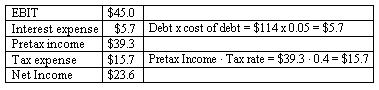### CFA Practice Question

There are 201 practice questions for this study session.

### CFA Practice Question

An analyst gathers the following data for a company (all data in \$MM):

• Total assets: \$380.
• Debt: \$114.
• Pretax cost of debt: 5%.
• Equity (26.6 million shares outstanding): \$266.
• Cost of equity: 14%.
• EBIT: \$45.
• Marginal tax rate: 40%

The residual income of the company using equity charge method is:
A. -\$12.8.
B. -\$10.4.
C. -\$13.6.
Explanation: We calculate net income first:Using the equity charge method, we calculate residual income:

Equity charge = Equity x cost of equity = \$266 x 0.14 = \$37.2.
Residual income = Net income - Equity charge = \$(13.6)